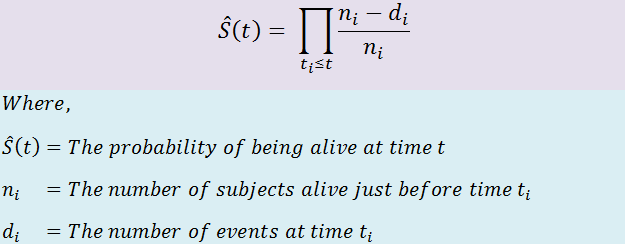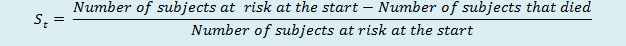# Survival Analysis on Conversion to Dementia

Survival Analysis on Conversion to Dementia

## Survival and hazard functions

1) Survival Function(S) 2) Hazard Function (H) To find the survival probability of a subject, we will use the survival function S(t), the Kaplan-Meier Estimator. The survival function is defined as the probability that an individual (subject) survives from the time origin (diagnosis of a disease) to a specified future time t. Please note that the time can be in various forms like minutes, days, weeks, months, or years. For example, S(200)=0.7 means that after 200 days, a subject’s survival probability is 0.7. In many deadly diseases, the survival probability decreases as the period increases. If the subject is alive at the end of an experiment, then that data will be censored.

The hazard probability, denoted by H(t), is the probability that an individual (subject) who is under observation at a time t has an event (death) at that time. For example, If h(200) = 0.7 means that after 200 days or on the 200th day, the probability of being dead is 0.7. One thing to keep in mind here is that the hazard function gives us the cumulative probability.

Notice that, in contrast to the survival function, which focuses on the survival of a subject, the hazard function gives us the probability of a subject being dead on a given time. We can note that higher survival probability and lower hazard probability is good for the subject’s health.

## Kaplan-Meier Estimator Theory and Example

The Kaplan–Meier estimator is a non-parametric statistic used to estimate the survival function (probability of a person surviving) from the lifetime data. In medical research, it is often used to measure the fraction of patients living for a specific time after treatment or diagnosis. For example: Calculating the amount of time(year, month, day) a particular patient lived after he/she was diagnosed with cancer or his treatment starts.

The probability of survival at time ti, which is denoted by S(ti), is calculated as follow:n a more generalized way, the probability of survival for a particular time is given by.Tags:

Categories:

Updated: